Unit 2 Math Integers

Unit 2 Study Guide

Integer Introduction Video

Determine the Opposite Video

Comparing Integers QUIZIZZ    Game Code: 647498

VOCABULARY

Integers
the set of whole numbers and their opposites
Negative Numbers
numbers that are less than zero
Positive Numbers
numbers that are greater than zero

GUIDENCE

In mathematics so far, you have learned about some different kinds of numbers. You learned about whole numbers, fractionsdecimals and percentages. In this Concept, you are going to learn about integers.

What is an integer?

An integer is a member of the set of whole numbers and their opposites.

To better understand integers, let’s first think about some real life situations where you might have seen integers used before.

Integers can be thought of as positive and negative numbers.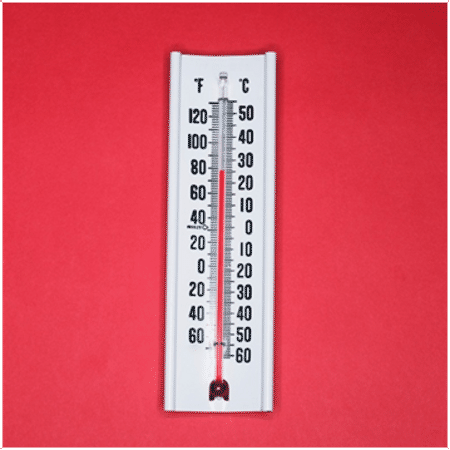If you look at this thermometer, you will see that the temperature is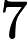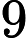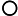This is 79 degrees above zero.

When we think about temperature, we use integers all the time. You might have heard someone talk about temperatures above or below zero. A temperature that is above zero is positive. A temperature that is below zero is negative.Integers are positive and negative whole numbers. Said another way, they are whole numbers and their opposites.

How can we write integers?

When writing an integer, we can use a + sign or a - sign. A + sign can be used for a number above zero or a positive number. A positive number can also be written without the + sign. Anegative number should be written using the - sign.

5 degrees below zero

The words “below zero” let you know that this integer is a negative number. Since it is five degrees below zero, we can write the integer negative five.

83 degrees

This temperature does not have a "-" sign in front of it, and does not say "below zero" either, it is a positive number. We write the integer positive eighty-three.

The answer is 83 or +83.

There are other real-life situations that use positive and negative numbers too.

One example is with money. A loss of money would be a negative integer. A gain of money is a positive integer.Jeff earned two hundred dollars working at the farm stand.

Jeff’s money is a gain. He “earned” it. That means that he had an increase in money and not a decrease. An increase or a gain is a positive integer. We can write the two hundred dollars as a positive integer.

The answer is $200.00 or +$200.00.

Sasha spent $45.00 at the clothing store. Since Sasha spent this money, it is a loss. Therefore, we can say that Sasha’s$45.00 is a negative integer. A negative integer can show a loss of money.

Solution: -100

Example C

A heat wave of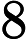Solution: +98

Practice Problems - Understanding Integers     (ANSWERS)

Understanding Positive and Negative Numbers - Independent Practice Worksheet

Understand A Rational Number As A Point    (ANSWERS)

Understand A Rational Number as A Point Independent Practice

Practice

Directions: Write an integer to represent each situation.

1. A loss of 20 points

2. A gain of 14 points

3. A profit of $20.00 4. A loss of$18.00

5. An elevation of 500 ft.

6. 200 feet below sea level

7. 8 degrees below zero

8. 78 degrees

9. A decrease of $68.00 10. An increase of$55.00

11. The dow did not rise or fall today.

12. The plane descended at a rate of 15%.

13. The submarine surfaced.

14. The cost and the earnings evened out.

15. The stock market had an overall rate of 18% above.